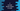# C program to convert binary values to octal## C program to convert binary values to octal:

In this post, we will learn how to convert a binary value to octal. The binary number system uses base 2 and the octal number system uses base 8.

We will write a program that will take a binary number as input and print the octal value for that binary number.

### How to convert a binary value to octal:

The easiest way to convert a binary value to octal is by converting the binary value to decimal and then converting the decimal value to octal.

• Convert binary to decimal
• Convert the decimal result to binary

So, we need to learn two different types of conversions. Let’s check how to do these:

#### Binary to decimal conversion:

The binary number system uses base 2 and the decimal number system uses base 10. If you want to convert a binary number to decimal, you need to follow the following steps:

• Start from right to left
• Multiply the rightmost digit with 2^n, n starts from 0. So, for the first digit, multiply it with 2^0, multiply the second digit with 2^1 etc.
• Add it to a sum variable. This variable is initialized as 0 and this will hold the final decimal value.
• Remove the rightmost digit.
• Follow the above steps until the number becomes 0.

For example, if you want to convert 1101 to decimal:

• decimal = 0
• 1101 -> Remove the last digit and multiply it with 2^0, add it to sum -> 0 + 1 * 2^0 = 1
• 110 -> Remove the last digit and multiply it with 2^1, add it to sum -> 1 + 0 * 2^1 = 1
• 11 -> Remove the last digit and multiply it with 2^2, add it to sum -> 1 + 1 * 2^2 = 5
• 1 -> Remove the last digit and multiply it with 2^3, add it to sum -> 5 + 1 * 2^3 = 13

So, 13 is the decimal representation of 1101.

#### Decimal to octal conversion:

We need to follow the following steps to convert a decimal to octal:

• Divide the decimal value by 8. store the remainder.
• Keep diving the value by 8 until it becomes 0.
• If we join the remainders in reverse order, it will be the octal value.

Let’s try to convert 200 to octal:

• 200/8 = 25 -> remainder: 0
• 25/8 = 3 -> remainder: 1
• 3/8 = 0 -> remainder: 3

So, 310 is the octal value of 200.

### C program:

Let’s write down the program in C. It will take the binary value as input from the user and print the octal result:

``````#include <stdio.h>

int binaryToDecimal(long long binary)
{
int decimal = 0;
int multiplier = 1;

while (binary != 0)
{
decimal += (binary % 10) * multiplier;
multiplier *= 2;
binary /= 10;
}

return decimal;
}

int decimalToOctal(int decimal)
{
int octal = 0;
int multiplier = 1;

while (decimal != 0)
{
octal += (decimal % 8) * multiplier;
decimal /= 8;
multiplier *= 10;
}

return octal;
}

int main()
{
long long binary;

printf("Enter the binary number: ");
scanf("%lld", &binary);

int decimal = binaryToDecimal(binary);
int octal = decimalToOctal(decimal);

printf("Octal value: %d\n", octal);

return 0;
}``````

Here,

• binaryToDecimal method is used to convert a binary to decimal.

• It created an integer variable decimal to hold the final decimal value and it is initialized as 0
• The multiplier is initialized as 2^0 i.e. 1
• The while loop runs until the value of the binary is not 0.
• Inside the loop, it gets the last digit of the binary number by using % 10 and multiplies it with multiplier. This value is added to decimal.
• The value of multiplier is updated and it removes the last digit.
• At the end of the method, it returns the decimal variable.
• decimalToOctal is used to convert a decimal value to octal.

• This method takes the decimal value as the parameter and returns its octal representation.
• The octal variable is to hold the final octal value and it is initialized as 0. The multiplier is initialized as 1.
• The while loop will run until the decimal value is greater than 0.
• On each step, it divides the decimal value by 8 and multiplies it with the multiplier. This value is added to the final octal value.
• It also updates the decimal value and multiplier on each step.
• At the end of this method, it returns the final octal value calculated.
• Inside main, it stores the binary value in a long long variable.
• It calls binaryToDecimal to convert the binary value to decimal and decimalToOctal to convert the decimal value to octal.
• The last line is printing the octal value calculated.

### Sample output:

If you run this program, it will print output as like below:

``````Enter the binary number: 10110
Octal value: 26``````